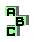NINECENTER   (5/6)

 r1896 centroid, altimedial triangles, concyclic points r1903 cevian triangle, radical center r1907 incenter, Euler line, incentric triangle, pedal triangle, isogonal points r1910 orthocenter, Euler line, altitudes r1913 orthocenter, circumcenter, Euler line, ninecircle r1917 orthocenter, isogonal points r1922 circumcenter, medial triangle, excentral triangle, Bevan point r1923 medial triangle, altitudes, orthologic triangles, point X(64) r1924 circumcenter, tangential triangle, Euler line, homothetic triangles r1937 orthocenter, cevian quotient, point X(52) r1943 incenter, excentral triangle, perspective, similar triangles r1944 incenter, circumcenter r1953 altitude feet, orthologic triangles r1954 altitude feet, orthologic triangles, Euler line, orthocenter, circumcenter, centroid r1957 circumcenter, Euler line r1958 incenter, circumcircle r1959 excenters, circumcircle r1960 excenters, circumcenter, Euler line r1982 incenter, excenters r1983 circumcenter, altitude feet, Euler line, point X(186) r1991 circumcenter, incenter, excircles r1993 circumcenter, incenter, concyclic points r2004 circumcenter, incenter, cevian quotient, radical center r2005 orthocenter, circumcenter, Euler line, cevian quotient, radical center r2008 circumcenter, Euler line, tangential triangle, homothetic triangles1 2 3 4 5 6